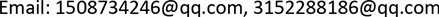1. 引言

2. 求解模型建立

M = ∑ i = 1 t q i = ∑ i = 1 t p i y i

Price list of unit cost and diameter of pipe materia

x1y1p1q1
x2y2p2q2
x3y3p3q3
xnynpnqn
xt

2.1. 一元线性回归模型

{ y = a + b x + ε E ( ε ) = 0 , D ( ε ) = σ 2

{ y i = a + b x i + ε i , i = 1 , 2 , ⋯ , n E ( ε i ) = 0 , D ( ε i ) = σ 2 , ε i > 0

Q = Q ( a , b ) = ∑ i = 1 n ε 2 = ∑ i = 1 n ( y i − a − b x i ) 2 (1)

Q ( a ^ , b ^ ) = Q min ( a , b ) (2)

{ ∑ i = 1 n ( y i − a ^ − b ^ x i ) = 0 ∑ i = 1 n x i ( y i − a ^ − b ^ x i ) = 0 (3)

{ a ^ = y ¯ − b ^ x ¯ b ^ = x y ¯ − x ¯   y ¯ x 2 ¯ − x ¯ 2 (4)

y = a ^ + b ^ x , y ¯ = b ^ ( x − x ¯ )

2.2. 一元多项式回归

f ( x ) = ∑ j = 0 m a j x j (5)

P min = ∑ i = 1 n [ f ( x i ) − y i ] 2 = ∑ i = 1 n ( ∑ j = 0 m a j x j − y i ) 2 (6)

∂ P ∂ a j = 2 ∑ i = 1 n ( ∑ k = 0 m a k x i k − y i ) x i j       ( j = 0 , 1 , 2 , ⋯ , m ) (7)

∂ P ∂ a j = 2 ∑ i = 1 n ( ∑ k = 0 m a k x i k − y i ) x i j = 0 (8)

∑ k = 0 m ( ∑ i = i n x i j + k ) a k = ∑ i = i n x i j y i ( j = 0 , 1 , 2 , ⋯ , m ) (9)

= [ c 0 c 1 ⋯ c m c 1 c 2 ⋯ c m + 1 ⋮ ⋮ ⋱ ⋮ c m c m + 1 ⋯ c 2 m ] × [ a 0 a 1 ⋮ a m ] = [ b 0 b 1 ⋮ b m ] (10)

{ c k = ∑ i = i n x i k , ( k = 0 , 1 , 2 , ⋯ , m ) b k = ∑ i = i n x i k y i , ( k = 0 , 1 , 2 , ⋯ , m ) (11)

f ( x ) = a 0 + a 1 x + ⋯ + a m x m = ∑ j = 0 m a j x j

2.3. 模型比较

f ( x ) = a 0 + a 1 x + ⋯ + a m x m ，

3. 数值算例

3.1. 具体求解过程

1) 做出散点图

2) 确定数学模型

① 一元线性回归模型。首先设 y i = a + b x i + ε i , i = 1 , 2 , ⋯ , n 再设

Q min = Q ( a , b ) = ∑ i = 1 n ε i 2 = ∑ i = 1 n ( y i − a − b x i 2 )

Price list of unit cost and diameter of pipe for graphite cast iron pipe

15096.9730
2100102.550
3150138.2770
4200186.2760
5250241.91120
6300295.47300
8400410.62150
10500563.8200

{ a ^ = y ¯ − b ^ x ¯ b ^ = x y ¯ − x ¯   y ¯ x 2 ¯ − x ¯ 2

{ a ^ = − 4.1816 b ^ = 53.0580

② 多项式回归模型

C = [ 8 39 255 39 255 1953 255 1953 16371 ] ， B = [ 2035.8 13367 104080 ]

C − 1 = [ 1.4610 − 0.5694 0.0452 − 0.5694 0.2673 − 0.0230 0.0452 − 0.0230 0.0021 ]

A = C − 1 B = [ 64.4780 18.0544 3.1994 ]

f ( x ) = 64.4780 + 18.0544 x + 3.1994 x 2

3.2. 误差对比分析

3.3. 模型代入求解

M = ∑ i = 1 t p i ( 64.4780 + 18.0544 x + 3.1994 x 2 ) 。